# What influences his choice?

In order for a building structure to be solid and reliable, it is necessary to carefully approach its calculations. For the truss system most often used ordinary wooden timber, the choice of which must be approached with full responsibility, as this affects the security and integrity of the whole house. It is best to calculate the cross-section of a timber using special programs, but such work is quite feasible even when using a number of formulas. Be sure to take into account wind and snow loads in the region, the characteristics of finishing materials and insulation.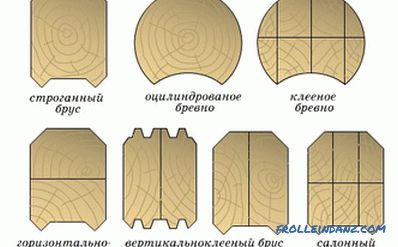Species of the section of the beam.

## What influences the rafter section?

To install a solid and reliable roofing system, to make the right choice, you need to pay attention to which timber is used for work. It is important to correctly calculate the truss system, for which the cross-section is of primary importance. It depends on the size of the bars, whether the rafters can withstand the weight of the roof.

When calculating, the following parameters are taken into account:Rafting section diagram.

1. The total weight of all used roofing materials.
2. The weight of the whole designed interior decoration, including attic and attic.
3. All calculated values ​​for rafter legs, beams.
4. Weather effects that affect the roof.

The following factors are additionally taken into account:

• spans between separate rafters;
• calculation of the section of rafters;
• pitch of mounted rafter legs;
• the form of the truss truss, features of fixing the rafters;
• other data that may influence the calculation.

To make calculations, it is best to use special programs or contact a specialist. Of course, there are a number of formulas that allow you to perform calculations yourself, but for the construction of a large and complex roof it is better to turn to professionals.

## Requirements for timber

In order for the rafter system to be solid and reliable, it is necessary to pay attention to the quality of the material itself when choosing a timber. For example, the moisture level should not be more than 20%. The bars must be treated with a special solution that will protect the material from rotting, damage by insects, open flame.

It must be remembered that the bar will be loaded. They can be permanent or temporary: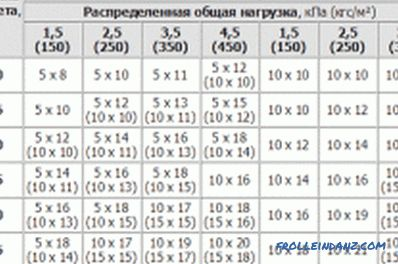1. The constants usually turn out to be the own weight of the entire truss structure, the used sheathing chosen for the cladding of the roofing material, insulation. This value is calculated for each material separately, after which the loads are summarized.
2. Temporary loads are divided into special rare, short-term, long-term. Among the special can be attributed to earthquakes. Short-term wind, snow, weight of people who are engaged in repair and other works on the roof. For long-term are all other types of loads that act for a certain time.

When calculating the cross section of the beam for rafters, it is important to take into account the snow load. For each region, this value is individual. To clarify the data you need to use special tables.

For calculations of all planned exact snow loads, use the following formula:

S = Sg * µ, where

1. Sg is the calculated exact value of the entire mass of snow, which accounts for every 1 m² of unpaved horizontal surface (should not be confused with the roof covering).
2. µ is the load transfer factor to the horizontal (or with a slope) surface of the roof. This coefficient is calculated taking into account the roof slope, it can take the following values:
• µ = 1 if the ramp has a slope of 25 degrees;
• µ = 0.7, if the slope of the slope is 25-60 degrees.

If the angle of the slope exceeds 60 degrees, the coefficient is not taken into account, since it has no significant effect on the section of the rafters.

In order for the system of rafters to be designed correctly, it is necessary to take into account wind loads, which have a significant effect on the structure.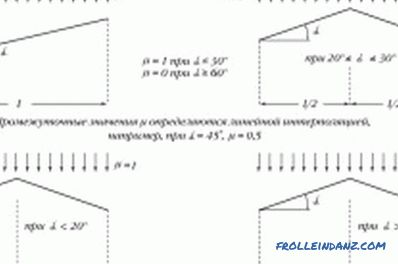Scheme of snow load on the rafters.

Do not underestimate them, as this can lead to disastrous consequences. To find out the average wind load on the roof system, you need to use a formula depending on the height readings (there are exact values) above the ground level:

W = Wo * k, where

• Wо - the standard value of wind load, which can be found in special directories for the region;
• k - change for wind pressure, which depends on height. Determined by tabular data.

The table itself is not very difficult to use; you just need to remember that the first column always shows the known constant values ​​for desert regions, forest-steppes, steppes, tundra, sea coasts, banks of reservoirs, lakes, rivers. In the 2nd column, all known values ​​are indicated for calculations related to urban areas, areas where obstacles have heights of 10 m. It is important to use wind direction data during calculations, as this can have a strong influence on the result obtained.

## Rules for calculating the beam cross-section

The cross-section of the truss system of any planned house depends on a number of parameters:

• length of one truss foot;
• step, s which will be mounted truss system;
• the calculated value of load indicators, which is typical for a specific area of ​​construction.

For calculations, it is necessary to use special data tables that contain ready-made values. For example, for the roof system of a house in the Moscow region, the following values ​​are applicable: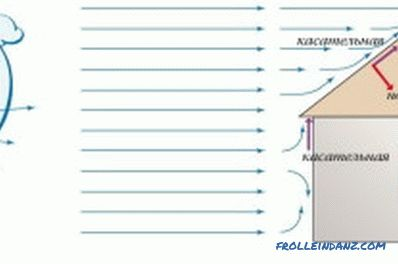• for mauerlat used wooden bars, the cross section of which will be 150 * 150 mm, 150 * 100 mm, 100 * 100 mm;
• for truss legs, diagonal ends are applied wooden bars with a cross section of 200 * 100 mm;
• products with a cross section of 200 * 100 mm, 150 * 100 mm, 100 * 100 mm;
• suitable for runs; a bar, section which is 150 * 50 m;
• for crossbars, bars must be used, the cross section of which is 200 * 100 mm, 150 * 100 mm;
• wooden bars with a cross section of 150 * 150 mm are used for racks , 100 * 100 mm;
• for the cornice, fillies, struts, suitable bars with parameters 150 * 50 mm;
• as a future frontal board and wooden sheet is used for filing, its section is 22 * ​​100 mm.

## An example of calculating a section of a wooden beam

An example of calculating rafters for a roof of a house shows what kind of material and quantity is needed, what section should be used. Initial data for the calculation:

1. The design load for the entire roof is 317 kg / m².
2. The standard used load in this used case is 242 kg / m²;
3. The angle of the skates is 30 degrees. In the horizontal planned projection, the length for one span is 450 cm, with L 1 = 300 cm, and L 2 = 150 cm.
4. The pitch of all mounted rafters is 80 cm.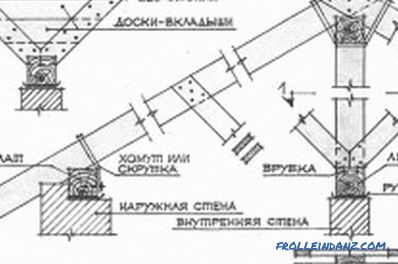Scheme of rafters.

Bolts will be used for fastening bolts to prevent nails from loosening the material. At the same time, for wood of the second grade, the resistance value will be 0.8 with a weakened section of the timber used: R izg = 0.8x130 = 104 kg / m².

Future load of the system on each linear meter of rafters:

• Qр = 317 * 0.8 = 254 kg / m;
• Qн = 242 * 0.8 = 194 kg / m.

If the slope for the roof is up to 30 degrees, then the rafter system will be considered flexible. The maximum moment of such bending is:

M = -qxx (L 13 + L 23 ) / 8x (L 1 + L 2 ), i.e. M = - 254 * (33 + 1.53) / 8 x (3 + 1.5) = - 215 kg / m.

The final value is M = -21500 kg / cm. Here the “-” sign used means that the bend will act in the opposite direction from the entire load applied to the work.

Next, you need to calculate the moment of resistance of rafters to bending force:

W = 21500/104 = 207 cm ³.

To make rafters, wooden rectangular bars with a width of 50 mm are usually used. On this basis, it is possible to obtain the height for the rafters with regard to the obtained data on the resistance:

H = √ (6x207 / 5) = 16 cm.

The section of the rafters is b = 5 cm, and the planned height is h = 16 cm. Referring to the standards that are governed by GOST, you can choose a wooden bar, which corresponds to the obtained parameters as much as possible: 175 * 50 mm. Such a value is used for a span of L 1 = 3 m. After this, it is necessary to calculate the rafter foot for the inertial moment:

J = 5 * 17.53 / 12 = 2233 cm³.

After that, you can get a value for deflection, which is also regulated by the standards: F OK = 300/200 = 1.5 cm.

Now you can calculate the deflection for regulatory loads :

F = 5 * 1.94 * 3004/384 * 100 000 * 2233, that is, you get the value F = 1 cm.

When reconciled with the data of the standard deflection, you can see that a value of 1 cm is less than the normative value of 1.5 cm. This indicates that the cross section of 175 * 50 mm has been chosen correctly, such material can be used for the construction of the roof truss system.

In order for the truss roof system used to be durable and reliable, able to withstand all planned loads, one should carefully approach the calculations for the cross section of the beam, which will be the main building material of the roof. For this purpose, a number of formulas are applied; during the calculations it is necessary to use special reference books with normative indicators. It is required to determine wind, snow loads and other important indicators.

•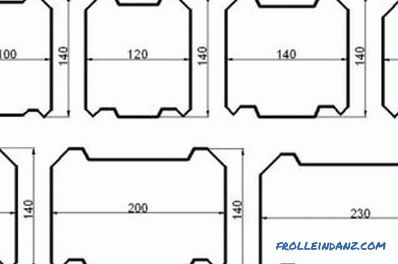What are the sizes of lumber?

How are sawn softwood sizes standardized? What is the width and thickness of building boards and boards of coniferous wood is recommended in the list of values ​​of GOST.

•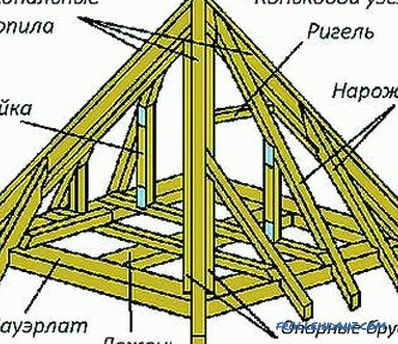Perform the calculation of roof rafters

The calculation of the rafters includes the determination of the pitch of the racks, the section of the timber. The configuration of the roof, the scheme of supporting the legs on the mauerlat, the joints in the ridge, the use of crossbars, racks are taken into account.

•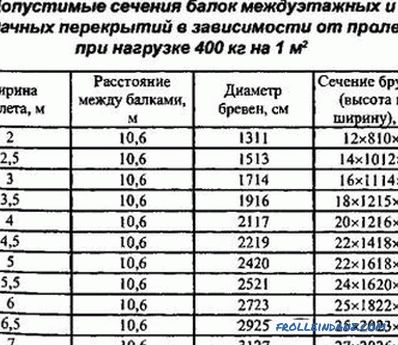How is the wood beam calculated?

Calculation of wooden beams at the design stage. Basic data for calculating the load. The load on the wooden structures, the cross section of the beams and the installation step. Example calculations